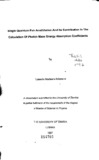## Single quantum pair annihilation and its contribution in the calculation of photon mass energy absorption coeefficients2012-08-28
##### Authors
Ndawana, Lutende Macleans
##### Abstract
This dissertation considers the single quantum pair annihilation of positrons with bound K-shell electrons in heavy atoms. It gives numerical calculations of the total cross section. By means of the method due to Basbas et a/.14, the binding-energy effect and its contribution to SQA cross sections is calculated. This calculation is done by use of a method suggested by Tseng and Pratt6. It is seen that at low positron energies the contribution due to binding-energy effect is significant. Recently Seltzer13 has shown that the contribution of single quantum pair annihilation cannot be neglected in the calculation of photon mass energy absorption coefficients. Seltzer13 included these contributions by means of an approximate analytical formula that tries to approximate the exact cross section calculations of Sheth and Swamy10 and of Broda and Johnson8. This approximate analytical formula used*by Seltzer13 is seen to be inconsistent with the behaviour of the cross section as a function of energy. In this work we propose an improved analytical formula that can be used in the above calculations.
##### Keywords
Special relativity(physics) , Nuclear Energy